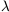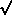2.3 Surveying the universe

The lookback time from the present day to an object at redshift z* is given by(36)

with H(a) given by (30). The age of the universe is obtained by taking the z* ->(t* -> 0) limit. For=M = 1, this yields the familiar answer t0 = (2/3) H0-1; the age decreases asM is increased, and increases asis increased. Figure (2) shows the expansion history of the universe for different values of these parameters and H0 fixed; it is clear how the acceleration caused byleads to an older universe. There are analytic approximation formulas which estimate (36) in various regimes [10, 11, 3], but generally the integral is straightforward to perform numerically.Figure 2. Expansion histories for different values ofM and. From top to bottom, the curves describe (M,) = (0.3, 0.7), (0.3, 0.0), (1.0, 0.0), and (4.0, 0.0).

In a generic curved spacetime, there is no preferred notion of the distance between two objects. Robertson-Walker spacetimes have preferred foliations, so it is possible to define sensible notions of the distance between comoving objects - those whose worldlines are normal to the preferred slices. Placing ourselves at r = 0 in the coordinates defined by (2), the coordinate distance r to another comoving object is independent of time. It can be converted to a physical distance at any specified time t* by multiplying by the scale factor R0 a(t*), yielding a number which will of course change as the universe expands. However, intervals along spacelike slices are not accessible to observation, so it is typically more convenient to use distance measures which can be extracted from observable quantities. These include the luminosity distance,(37)

whereL is the intrinsic luminosity and F the measured flux; the proper-motion distance,(38)

where u is the transverse proper velocity andthe observed angular velocity; and the angular-diameter distance,(39)

where D is the proper size of the object andits apparent angular size. All of these definitions reduce to the usual notion of distance in a Euclidean space. In a Robertson-Walker universe, the proper-motion distance turns out to equal the physical distance along a spacelike slice at t = t0:(40)

The three measures are related by(41)

so any one can be converted to any other for sources of known redshift.

The proper-motion distance between sources at redshift z1 and z2 can be computed by using ds2 = 0 along a light ray, where ds2 is given by (2). We have(42)

where we have used (5) to solve for R0 = 1 / (H0(|k0|)), H(a) is again given by (30), and ``sinn(x)'' denotes sinh(x) whenk0 < 0, sin(x) whenk0 > 0, and x whenk0 = 0. An analytic approximation formula can be found in . Note that, for large redshifts, the dependence of the various distance measures on z is not necessarily monotonic.

The comoving volume element in a Robertson-Walker universe is given by(43)

which can be integrated analytically to obtain the volume out to a distance dM:(44)

where ``sinn'' is defined as below (42).